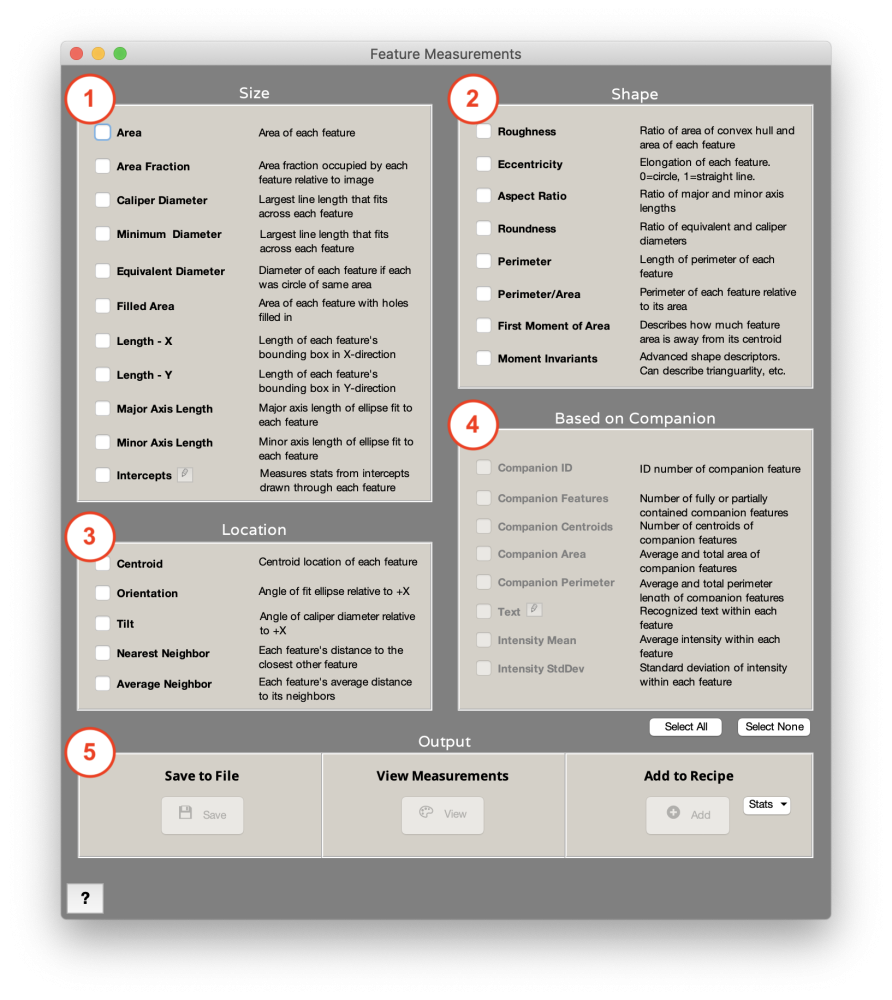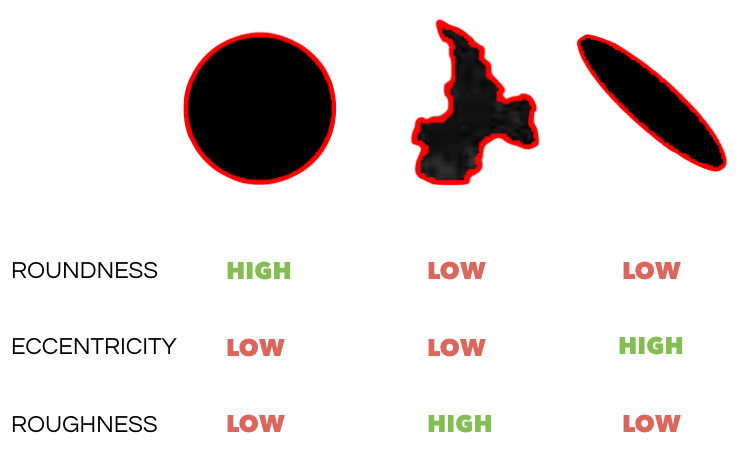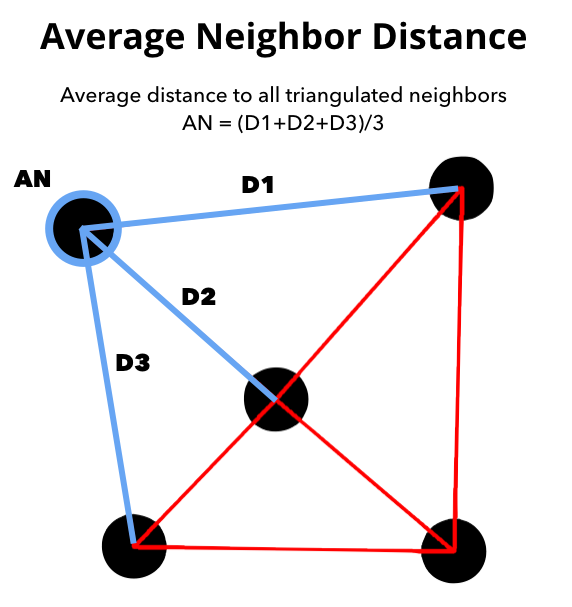### Measurements > Feature Measurements

Feature Measurements allows you to select a number of types of measurements you would like to generate based on the features you have in your image.
me### 1. Size

• Area: Area of each feature.
• Area Fraction: Area fraction occupied by each feature relative to entire image.
• Caliper Diameter: Largest line length that fits within each feature.
• Minimum Diameter: Smallest line length that fits between two parallel lines tangential to each feature.
• Equivalent Diameter: Diameter of each feature if each was a circle of the same area.
• Filled Area: Area of each feature with holes filled in.
• Length – X: Length of each feature’s bounding box in the X-direction.
• Length – Y: Length of each feature’s bounding box in the Y-direction.
• Major Axis Length: Major axis length of ellipse fit to each feature .
• Minor Axis Length: Minor axis length of ellipse fit to each feature .
• Intercepts: Measures statistics from intercept length recorded from each feature.
- Through Centroid: Draw each line through each feature’s centroid.
- Along Vector: Draw each line along the same direction through each feature.

### 2. Shape

• Roughness: Ratio of area of tightest-fitting convex hull and area of each feature.
• Eccentricity: Describes how elongated or circular each feature is. 0 is a perfect circle. 1 is a straight line. Eccentricity is calculated from the ellipse fit to each feature as sqrt(-1([minor axis length]/[major axis length])^2) )
• Aspect Ratio: Ratio of major and minor axis lengths, as defined by the ellipse fit to each feature .
• Roundness: Ratio of equivalent and caliper diameters.
• Perimeter: Length of perimeter of each feature.
• Perimeter/Area: Perimeter of each feature relative to its area.
• First Moment of Area: Describes how much area is extended away from each feature’s centroid. Calculated as the summation of (area x distance from centroid) over all pixels in the feature.
• Moment Invariants: High-order moments which describe different shape properties of each feature [2,3].### 3. Location

• Centroid: Centroid location of each feature.
• Orientation: Angle of the ellipse fit to each feature with respect to the positive X-axis. Positive angles are clockwise rotations and negative counterclockwise.
• Tilt: Angle of the caliper diameter of each feature with respect to the positive X-axis. Positive angles are clockwise rotations and negative counterclockwise.
• Nearest Neighbor: Each feature’s distance to the closest other feature, calculated from the features’ centroids.
• Average Neighbor: Each feature’s average distance to its neighbors, as defined by the features’ Delaunay triangulation. Triangulation and distance are both calculated from the features’ centroids.### 4. Based on Companion

Requires Companion Image Each layer’s Companion Image is determined independently from the recipe, as the latest Companion Image set before the layer step.

• Companion ID: ID number of the feature in the Companion Image that contains the centroid of each feature being measured.
• Companion Features: Number of features in the Companion Image which are within or in contact with each feature in the Current Image.
• Companion Centroids: Number of centroids in the Companion Image within each feature in the Current Image.
• Companion Area: Average and total area of features in the Companion Image within each feature in the Current Image.
• Companion Perimeter: Average and total perimeter length of features in the Companion Image within each feature in the Current Image.
• Text: Recognizes text within each feature. Set the binary mask or grayscale image as the companion. The Current Image should the the bounding box of the text, with the major axis running parallel to the text orientation.
- Edit: Allows the text search to be limited to just numbers
- Sample Image Sample Recipe
• Intensity Mean: Average grayscale intensity in the latest Companion Image within each feature in the Current Image.
• Intensity StdDev: Standard deviation of grayscale intensity in the latest Companion Image within each feature in the Current Image.

### 5. Output

• Save to File: Saves the selected feature measurements to a tab-delimited .txt file.
• View Measurements: View the selected measurements as a list, histogram, and image overlay (in Color by Measurements only). For measurements made on one layer, they will be viewed in our Color by Measurements tool, while measurements made on several or all layers will be viewed in our Histogram of Measurements tool.
• Add to Recipe: Adds the selected summary statistics to the recipe. Options are Mean, Standard Deviation, Max, Min, and Sum.

## References

 Ellipse is fit to the feature as the ellipse with the same second moments as the feature. Second moments are calculated as:
uxx = sum(x^2)/N + 1/12
uyy = sum(y^2)/N + 1/12
uxy = sum(x*y)/N

where x is the x coordinate of each pixel in the feature, y is the y coordinate of each pixel, and N is the number of pixels.

The major and minor axes are calculated as:

common = sqrt((uxx – uyy)^2 + 4*uxy^2)
MajorAxisLength = 2*sqrt(2)*sqrt(uxx + uyy + common)
MinorAxisLength = 2*sqrt(2)*sqrt(uxx + uyy – common)

 Rosin, Paul L. 2003. “Measuring Shape: Ellipticity, Rectangularity, and Triangularity.” Machine Vision and Applications 14 (3): 172–184.

 MacSleyne, J. P., Simmons, J. P., & De Graef, M. (2008). On the use of 2-D moment invariants for the automated classification of particle shapes. Acta Materialia, 56(3), 427–437.

Need more help with this?
Chat with an expert now ››